# Fibonacci Number Patterns

Here, for reference, is the Fibonacci Sequence:

1, 1, 2, 3, 5, 8, 13, 21, 34, 55, 89, 144, 233, 377, 610, 987, …

We already know that you get the next term in the sequence by adding the two terms before it. But let’s explore this sequence a little further.

First, let’s talk about divisors. Let me ask you this: Which of these numbers are divisible by 2?

1, 1, 2, 3, 5, 8, 13, 21, 34, 55, 89, 144, 233, 377, 610, 987, …

Every third number, right? And 2 is the third Fibonacci number. Okay, maybe that’s a coincidence. How about the ones divisible by 3?

1, 1, 2, 3, 5, 8, 13, 21, 34, 55, 89, 144, 233, 377, 610, 987, …

Every fourth number, and 3 is the fourth Fibonacci number. Okay, that could still be a coincidence. What about by 5?

1, 1, 2, 3, 5, 8, 13, 21, 34, 55, 89, 144, 233, 377, 610, 987, …

Every fifth number. And 8?

1, 1, 2, 3, 5, 8, 13, 21, 34, 55, 89, 144, 233, 377, 610, 987, …

Every sixth number. Now does it look like a coincidence? In fact, it can be proven that this pattern goes on forever: the nth Fibonacci number divides evenly into every nth number after it! Cool, eh?

Okay, now let’s square the Fibonacci numbers and see what happens.

$1^2 = 1$

$1^2 = 1$

$2^2 = 4$

$3^2 = 9$

$5^2 = 25$

$8^2 = 64$

$13^2 = 169$

The Fibonacci sequence is all about adding consecutive terms, so let’s add consecutive squares and see what we get:

$1+1=2$

$1+4=5$

$4+9=13$

$9+25=34$

$25+64=89$

$64+169=233$

We get Fibonacci numbers! In fact, we get every other number in the sequence!

So that’s adding two of the squares at a time. What happens when we add longer strings? Three or four or twenty-five?

$1+1+4=6$

$1+1+4+9=15$

$1+1+4+9+25=40$

$1+1+4+9+25+64=104$

$1+1+4+9+25+64+169=273$

The resulting numbers don’t look all that special at first glance. But look what happens when we factor them:

$6=2\cdot3$

$15=3\cdot5$

$40=5\cdot8$

$104=8\cdot13$

$273=13\cdot21$

And we get more Fibonacci numbers – consecutive Fibonacci numbers, in fact. Okay, that’s too much of a coincidence. Let’s ask why this pattern occurs. We have squared numbers, so let’s draw some squares.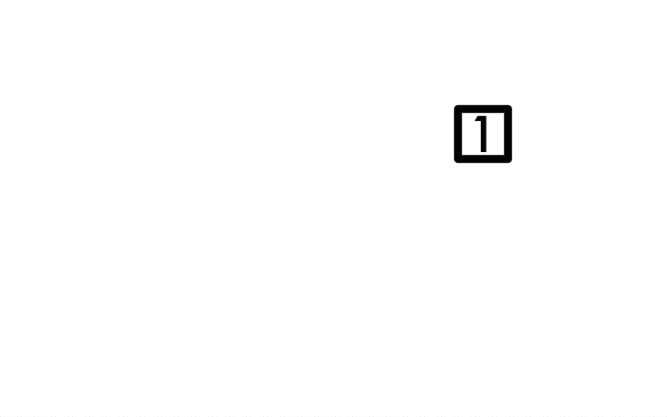This is a square of side length 1. Its area is 1^2 = 1. We draw another one next to it: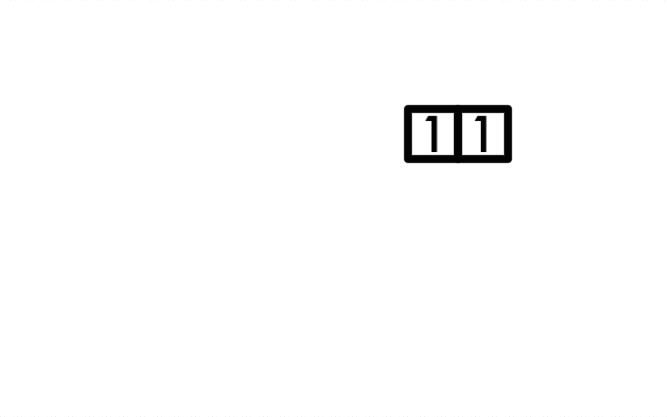Now the upper edge of the figure has length 1+1=2, so we can build a square of side length 2 on top of it: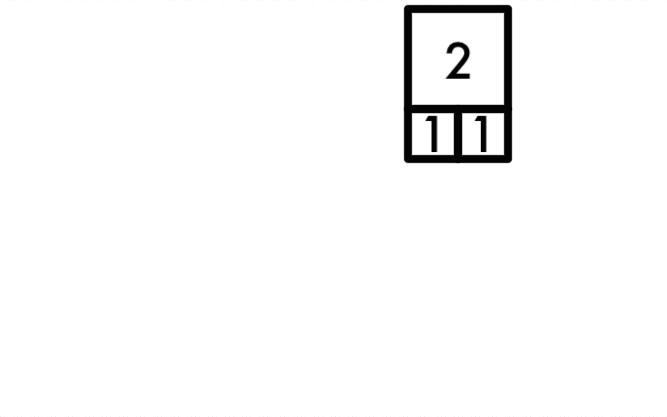Now the length of the rightmost edge is 1+2=3, so we can add a square of side length 3 onto the end of it.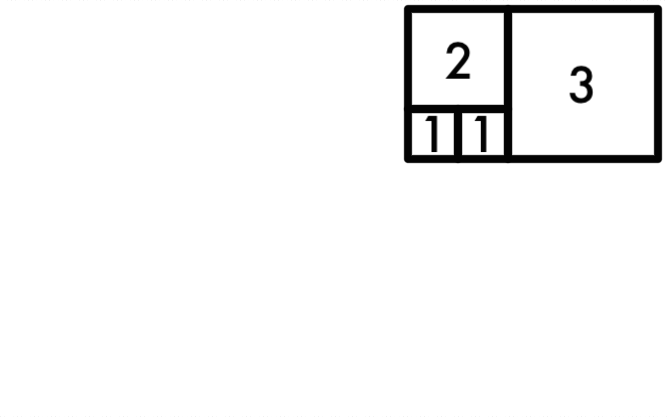Now the length of the bottom edge is 2+3=5: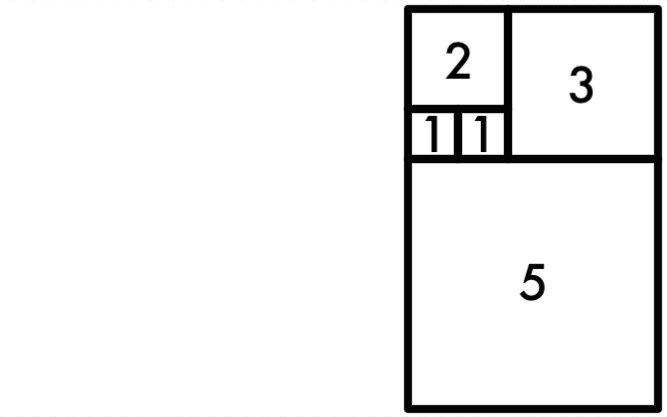And that makes the leftmost edge 3+5=8: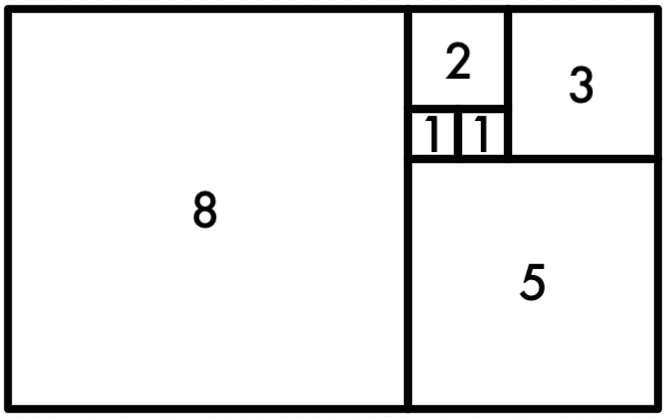And we can do this because we’re working with Fibonacci numbers; the squares fit together very conveniently. We could keep adding squares, spiraling outward for as long as we want. But we’ll stop here and ask ourselves what the area of this shape is. Well, we built it by adding a bunch of squares, and we didn’t overlap any of them or leave any gaps between them, so the total area is the sum of all of the little areas: that’s $1^2 + 1^2 + 2^2 + 3^2 + 5^2 + 8^2$. But the resulting shape is also a rectangle, so we can find its area by multiplying its width times its length; the width is $8$, and the length is $5 + 8 = 13$…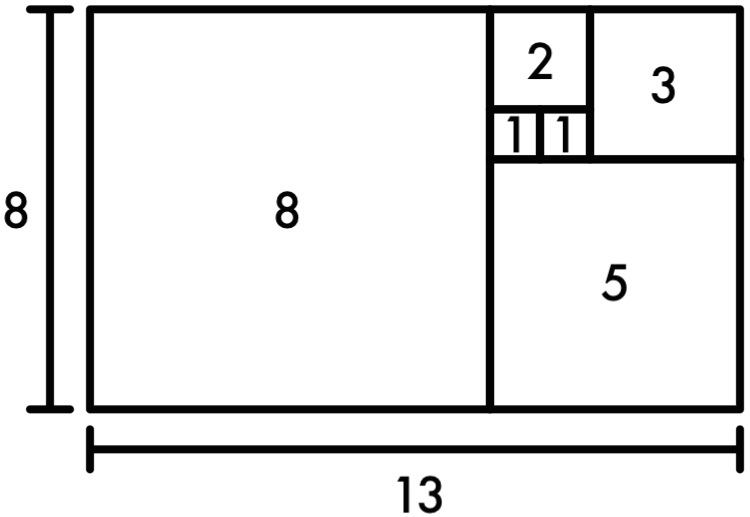… and the area becomes a product of Fibonacci numbers. That’s a wonderful visual reason for the pattern we saw in the numbers earlier! If we generalize what we just did, we can use the notation that $F_n$ is the $n$th Fibonacci number, and we get:

$$F_1^2 + F_2^2 + F_3^2 + \ldots + F_n^2 = F_n \cdot F_{n+1}$$

One more thing: We have a bunch of squares in the diagram we made, and we know that quarter circles fit inside squares very nicely, so let’s draw a bunch of quarter circles: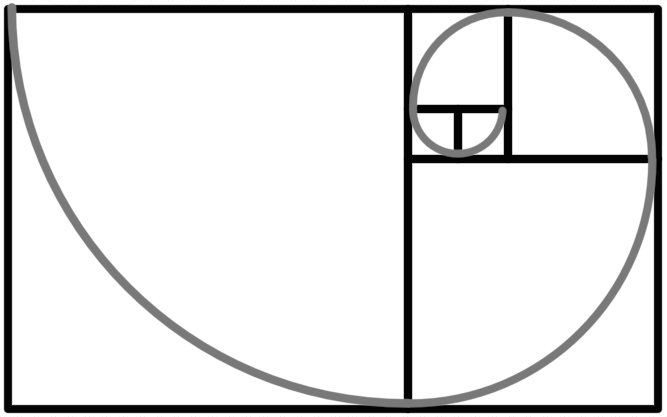And presto! We have what’s called a Fibonacci spiral. It’s a very pretty thing. That’s not all there is to the story, though: read more at the page on Fibonacci in nature.

What’s more, we haven’t even covered all of the number patterns in the Fibonacci Sequence. In particular, there’s one that deserves a whole page to itself